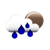### nPr Calculator or Permutation Calculator

 Example: 3P1 = 3! / (3 - 1)! = 3 n(objects):     r(sample):

#### What is permutation(nPr)?

In maths, nPr is used to find out the number of ways to choose r objects (subset) from n number of objects (larger set), which the order of choosing the objects should also be considered. For example, if i have 3 cards J,Q,K. I want to choose 2 out of them. How will i choose? I can either choose J,Q or Q,J or Q,K or K,Q or J,K or K,J. so it means that I have 6 ways to choose.

In that case, n = 3(larger set) and r = 2(subset). The combinations with order is: 3P2, which is 6.

#### The nPr Formula or Permutation Formula

You can use the nPr formula to find the nPr value:

nPr
=
n! / (n-r)!

Example:

4P2
=
4! / (4-2)!
= 12

#### References

More references for nPr

#### Local Weather Status

Ashburn, United States
9th December, 2019 Monday
Light Rain6.67 - 9.44
Humidity: 93 %
Wind: 3.6 km/h